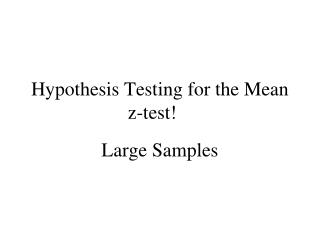DownloadDownload PresentationHypothesis Testing for the Mean z-test!

Hypothesis Testing for the Mean z-test!

Télécharger la présentationHypothesis Testing for the Mean z-test!

- - - - - - - - - - - - - - - - - - - - - - - - - - - E N D - - - - - - - - - - - - - - - - - - - - - - - - - - -
Presentation Transcript

1. Hypothesis Testing for the Mean z-test! Large Samples

2. Decision Rule Based on P-value • If • If • Examples: • The P-value for a hypothesis test is P = 0.023. What is your decision if the level of significance is • ? • ? Reject the null hypothesis Fail to reject the null hypothesis

3. Finding the P-value for a Hypothesis Test After determining the hypothesis test’s standardized test statistic and the test statistic’s corresponding area, do one of the following to find the P-value. • For a left-tailed test, P = (Area in left tail). • For a right-tailed test, P = (Area in right tail) • For a two-tailed test, P = 2(Area in tail of test statistic)

4. Example #1 1. Find the P-value for a left-tailed hypothesis test with a test statistic of z = -1.48. Decide whether to reject H0 if the level of significance is = 0.05. Solution: P(z = -1.48) = 0.069 Since p > 0.05, fail to reject the H0. Evidence at the 5% level supports the null hypothesis. P = 0.069

5. Example #2 Find the P-value for a two-tailed hypothesis test with a test statistic of z = 2.31. Decide whether to reject H0 if the level of significance is = 0.01. Solution P(z > 2.31) = 0.0104 P= 2(Area in both tails) = .0219 Fail to reject the H0, there is enough evidence to support the H0 at the 1% level since p > 0.01. P = 0.0104 P = 0.0104

6. Steps for z-Test

7. Changing the level of significance to a z-score in order to find the critical value. • Specify the level of significance • Decide whether the tail is left tailed, right tailed, or two tailed. • Find the critical value(s) z0. • Left tailed, find the z-score that corresponds to an area of • Right tailed, find the z-score that corresponds to an area of 1 – • Two tailed, find the z-scores that correspond to and 1 -

8. NOW YOU GET TO DO PRACTICE 7.2!